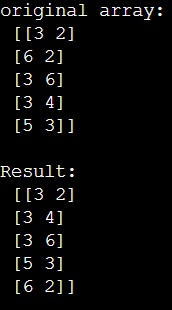# How to sort a 2D NumPy array by multiple axes?

Learn, how to sort a 2D NumPy array by multiple axes in Python? By Pranit Sharma Last updated : April 03, 2023

Suppose that we are given a 2D numpy array of shape N, 2 (with fixed two columns and multiple rows) where each row holds two values (x and y coordinates) and we need to sort this array in such a way that the point is ordered by x-coordinate and then by y in cases where the x coordinate is the same.

## NumPy 2D Array : Perform Sorting by multiple axes

To sort a 2D NumPy array by multiple axes, we use numpy.lexsort() method. It performs an indirect stable sort using a sequence of keys. In our case, we have x and y coordinates as the sequence of keys. We will pass the second column values and then the first column values as the pair of keys as a parameter.

Let us understand with the help of an example,

## Python code to sort a 2D NumPy array by multiple axes

```# Import numpy
import numpy as np

# Creating a numpy array
arr = np.array([(3, 2), (6, 2), (3, 6), (3, 4), (5, 3)])

# Display original array
print("original array:\n",arr,"\n")

# Sorting the array
res = np.lexsort((arr[:,1],arr[:,0]))

# Display result
print("Result:\n",arr[res])
```

### Output